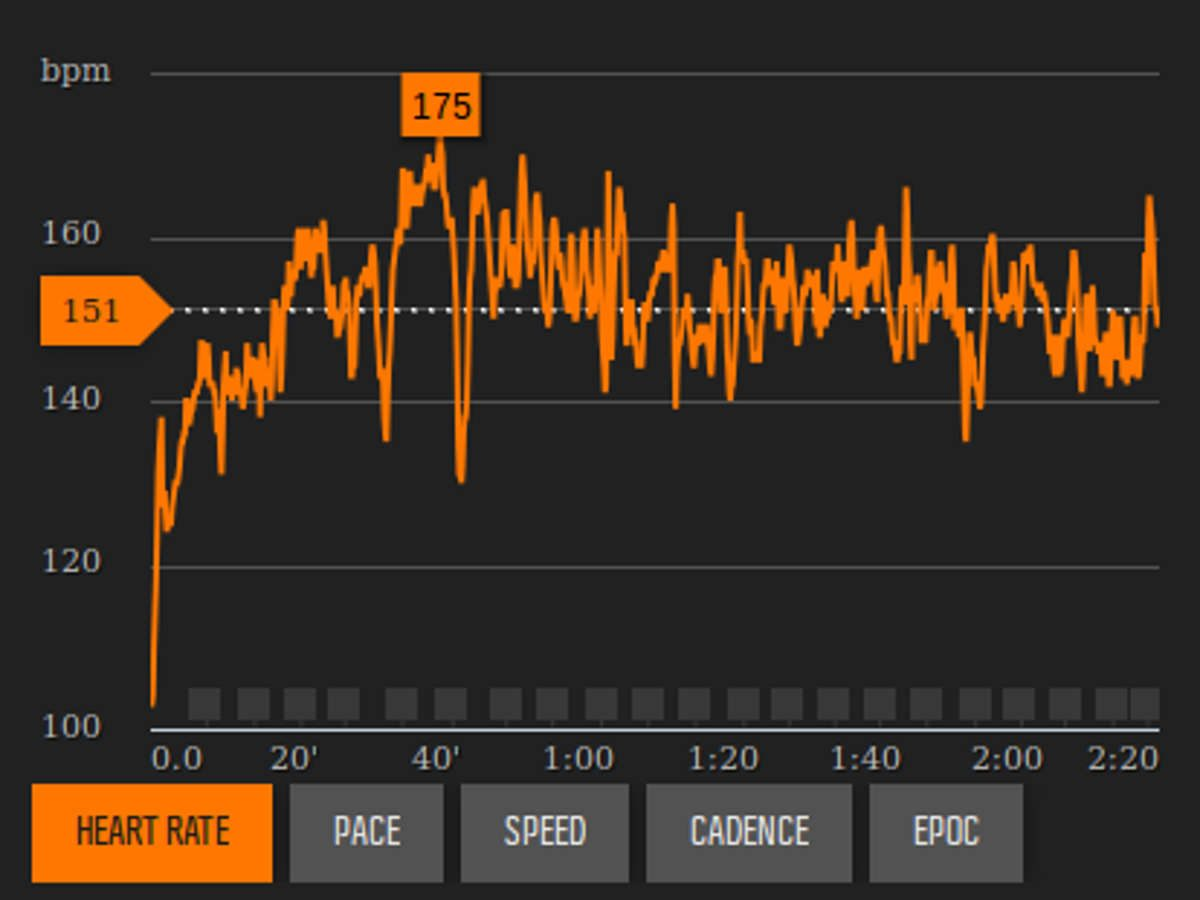# The Karvonen Formula to Calculate Heart Rate Zones

Step by step guide to calculate your heart rate zones using the Karvonen formula. There is an example included – and a calculator.The Karvonen formula is one of the bests that can be used to define your heart rate zones and anaerobic threshold. It is based on the heart rate reserve, so it takes into account both your resting heart rate and maximum heart rate. Therefore it gives a much better result than the formulas that use only the maximum heart rate.

Calculating your heart rate zones using the Karvonen formula is very easy and it takes only a few minutes. I will show you what steps you have to follow and at the end there is an example included, too.

To use this formula you need to know your resting and maximum heart rates.

After the calculation you will have your heart rate zones and anaerobic threshold that you can apply to your training — the exact meaning and application of the zones and threshold varies with every training plan.

## Requirements

To calculate your heart rate zones you will need two numbers, and basic math skills:

## Heart Rate Reserve

The Karvonen formula uses the heart rate reserve — this is a number, the size of the range between your resting heart rate and maximum heart rate. To calculate it all you need to do is to subtract the resting heart rate from the maximum heart rate.

HRreserve = maxHR – restingHR

You need to calculate this number as it is used to calculate the heart rate zones.

## Target Heart Rate

To calculate the heart rate zones all you need to do is to add your resting heart rate to a certain percentage the heart rate reserve. This is the formula:

targetHR = HRreserve x intensity% + restingHR

The intensity number is defined by the Karvonen Formula.

## Karvonen Heart Rate Zones

The Karvonen formula defines the following five zones, using two numbers two define each zone — the lower and the higher end of it:

• Zone1: 50% – 60% intensity
• Zone2: 60% – 70% intensity
• Zone3: 70% – 80% intensity
• Zone4: 80% – 90% intensity
• Zone5: 90% – 100% intensity

The first three zones are aerobic, the last two are anaerobic. Your anaerobic threshold is around the 80% intensity.

After you apply these intensity numbers to the target heart rate formula you will have your heart rate zones.

## Example

The last time I have measured my heart rate was about two years ago. I will use those numbers to show you an example. Don’t forget that everyone has different resting and maximum heart rates, so use your heart rates when doing the calculations!

• my resting heart rate: 42
• my maximum heart rate 201

Calculating the heart rate reserve:

HRreserve = 201 – 42 = 159

Calculating the zones:

159 x 50% + 42 = 80 + 42 = 122
159 x 60% + 42 = 95 + 42 = 137
159 x 70% + 42 = 111 + 42 = 153
159 x 80% + 42 = 127 + 42 = 169
159 x 90% + 42 = 143 + 42 = 185
159 x 100% + 42 = 159 + 42 = 201

All I had to do is to apply the heart rate reserve, the intensity and the resting heart rate to the formula. The only number that changes is the intensity.

And now the heart rate zones can be defined:

• Zone1: 122 – 137
• Zone2: 137 – 153
• Zone3: 153 – 169
• Zone4: 169 – 185
• Zone5: 185 – 201

Done!

## Calculator

You can use the calculator to define your heart rate zones.